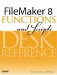# Pi

Category: Trigonometric

 Syntax: Pi

Parameters: None

Description:

Returns the value of the trigonometric constant Pi, which is approximately 3.1415926535897932. Pi is defined as the ratio of the circumference to the diameter of any circle.

Pi is most often used in conjunction with other trigonometric functions, such as Sin(), Cos(), and Tan(), which each require an angle measured in radians as a parameter. There are 2xPi radians in 360 degrees.

Examples:

Function

Results

Pi / 2

Returns 1.5707963267948966.

SetPrecision (Pi; 25)

Returns 3.1415926535897932384626434.FileMaker 8 Functions and Scripts Desk Reference
ISBN: 0789735113
EAN: 2147483647
Year: 2004
Pages: 352